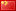## 16级程序设计基础实践第一次月考

1463 1 2016年10月27日

0 0 投票数

16级程序设计基础实践第一次月考
Begin Time: 2016-10-27 13:00:00
End Time: 2016-10-27 14:45:00
Judge: By File

A Lab04_01 39.52%(132/334)
B Lab04_02 7.88%(32/406)
C Lab04_03 8.55%(33/386)
D Lab04_04（选做） 25.71%(18/70)

Problem A:Lab04_01
Time Limit:1000MS Memory Limit:30000KB
Description

Input

Output

Sample Input
1 1
Sample Output
1
1
0

#include <stdio.h>
#include <stdlib.h>

int main() {
int a,b;
scanf("%d%d",&a,&b);
printf("%d\n",a||b);
printf("%d\n",a|b);
printf("%d\n",a^b);
return 0;
}

Problem B:Lab04_02
Time Limit:1000MS Memory Limit:30000KB
Description

Input:
3
Output:
2,1:16
Note:

Input

Output

Sample Input
0
Sample Output
0,0:32

#include <stdio.h>
#include <stdlib.h>
int gcd(int a, int b) {
if (b==0) {
return a;
} else {
return gcd(b,a%b);
}
}

int main() {
unsigned int num;
int i=0,count=0,a= {0};
scanf("%ud",&num);
while (num>0) {
a[i]= num % 2;
num = num / 2;
i++;
}
num=i;
while (i>=0) {
if (a[i]==1) count++;
i--;
}

if (count==0) {
printf("%d,%d:%d\n",0,0,32);
} else {
int div =gcd (count,32);
printf("%d,%d:%d\n",count,count/div,32/div);
}
return 0;
}

1、数组a开到了100，有点浪费了。

2、这里的输入数字num采用的是unsigned int。

3、计数的过程与前一篇略有差异，这里采用的是从数组最后一位往前的方法。

4、由于任何数都是0的因数，所以0与32的最大公约数是32，因此需要单独考虑count为0的情形。

5、约分到最简，就是分子分母同时除以最大公约数。

6、输出结果需要有换行符。

7、变量一定要记得初始化，这里都是赋初值0。

Problem C:Lab04_03
Time Limit:1000MS Memory Limit:30000KB
Description

Input:
The C
programming language
Output:
2
Note：

Input

Output

Sample Input
This is a exmaple
there are SOME Books
Sample Output
3

#include <stdio.h>
#include <stdlib.h>

int main() {
char ch;
int isnew=1,count=0;

while (scanf("%c",&ch)==1) {
if (ch>='A' && ch <='Z') {
if (isnew) {
count++;
isnew=0;
}
} else {
isnew=0;
if (ch==' '|| ch=='\n'|| ch=='\t') {
isnew=1;
}
}
}
printf("%d\n",count);
return 0;
}


1、因为空格有一个或多个，所以不能单纯地以flag=!flag这种方法来更改逻辑值。

2、判断是否文件结束仍旧采用的是scanf的返回值。

3、

if (ch>='A' && ch <='Z') { //如果这是大写字母
if (isnew) { //判断是不是一个新单词的开头
count++; //如果是，就让计数器加1
isnew=0; //并且把isnew设置为false
}

4、在else的部分，有一句isnew=0。这里详细说说这句话的含义。

else {  //否则（也就是不是大写字母）
isnew=0; //一个字符不是大写字母//不论是小写字母还是其他的//都认为之后遇到的不是一个新单词//把isnew设置为false//即便是空格，也先这样处理，之后再改
if (ch==' '|| ch=='\n'|| ch=='\t') {
isnew=1;
//如果是空格，那么之后遇到的就应该是新一个单词了
}
}

5、输出最后要有换行符。

Problem D:Lab04_04（选做）
Time Limit:1000MS Memory Limit:30000KB
Description

Note：

（注：图略）
Input

Output

Sample Input

Sample Output

#include <stdio.h>
#include <stdlib.h>
#include <math.h>

double f(double x) {
return (2*pow(x,3)-4*pow(x,2)+3*x-6);
}
//原函数

double f1(double x) {
return (6*pow(x,2)-8*x+3);
}
//导数

int main() {
double x=1.5;
//从1.5开始试
while (fabs(f(x))>0.00001) {
//精度要求
x=(-(f(x)))/(f1(x))+x;
//N-R定理
}
printf("%0.3f\n",x);
//场宽（域宽）
return 0;
}

//特别注意，绝对值是fabs()，不是abs()



#include<stdio.h>
#include<stdlib.h>
#include<math.h>
int main()
{
int p,i,n,t,x,y,u;
scanf("%d",&p);
i=0;
n=0;
t=1;
while(n<32)
{
if((p&t)==t)
{
i++;
}
else
;
n++;
t=pow(2,n);
}
if(i==0||i==1)
{
y=i;
u=32;
}
else if(i%2!=0)
{
y=i;
u=32;
}
else
{
for(x=2; i>=x;)
{
if(i%x==0)
{
y=i/x;
u=32/x;
}
x=2*x;
}
}
printf("%d,%d:%d\n",i,y,u);
return 0;
}


<

pre class=”lang:xhtml decode:true”>数组
a[]
arr[]
array[]
data[]
e[]
m[]
mat[]
mtx[]
matrix[]

ans

ave
avg
average

a
b

c
cnt
count
counter
tot
total

ch

dist

disk

dfs

dividend

divisor

dp

edt
edit
editor

f
flag

isXXX

isnew
isprime
isexist
isopen
isfinished
ismore

f
fac
fact
fct
func

g
grp
gpe
group

gcd

i
ii
j
jj
k
kk

loc
location

len
length

m
n
num

max

min

mgmt
management

p
pt
lnk

qs
qsort
q_sort
quicksort

rest

ret

s
st
str

sum

t
tmp
temp

t
time
mmt
moment

x
y
z

0 0 投票数

1条留言（可选）如果您也有个人网站，不妨分享一下

1 评论2016年10月27日 20:28